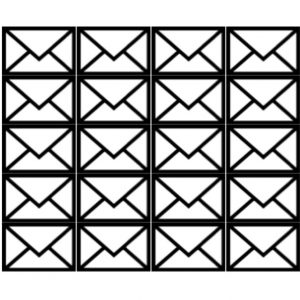In front of you there are 20 envelopes.

Eight of the envelopes each contain 5 blue and 3 red sheets of paper.

The other 12 envelopes each contain 6 blue and 2 red sheets of paper.

You choose one envelope at random. Then you choose a sheet of paper from it at random. What is the probability that this sheet of paper is red?

Are the chances of drawing a red or a blue card the same? If there are not equal numbers of red and blue cards in all the envelopes could you change the numbers of each colour to make the chances equal?

Suppose this is a gambling game. Put blue cards and red cards in 20 identical envelopes as described. Choose an envelope at random then choose a card. If you draw a red card you win. If it’s blue you lose. Is it a fair game? Play the game repeatedly as a probability experiment, and keep score. Does the experimental probability match the theoretical probability?

What happens if you make the total numbers of red and blue cards the same, say 4 blue and 1 red in 8 envelopes and 1 blue and 3 red in 12 envelopes making a total of 44 red and 44 blue cards? Does this make it a fair game? If so why, if not why not?